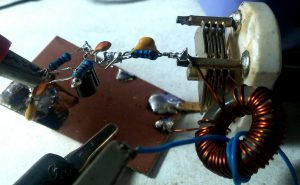# Factors affecting the frequency stability of an oscillator

The ability of an oscillator to maintain a constant frequency of oscillation is called as frequency stability.

There are following factors affect the frequency stability which are given below,

• Operating point of the active device: The Q-point of the active device i.e., transistor is so chosen as to confine the circuit operations on the linear portion of its characteristic. Operations on non-linear portion vary the parameters of the transistor which, in turn, affects the frequency stability of the oscillator.
• Inter-element capacitances: Any changes in the inter element capacitances of a transistor particularly the collector to emitter capacitances cause changes in the oscillator output frequency, Thus affecting its frequency stability. The effect of changes in inters element capacitances, can be neutralized by adding a swamping capacitor cross the offending elements the added capacitance being made part of the tank circuit.
• Power supply: Changes in the DC operating voltages applied to the active device shift the oscillator’s frequency. This problem can be avoided by using regulated power supply.
• Temperature variations: Variations in temperature cause changes in transistor parameters and also changes the values of resistors, capacitors and inductors used in the circuit. Since such changes take place slowly, they cause a slow change in the oscillator output frequency.
• Output load: A change in the output load may cause a change in the Q-factor of the LC tuned circuit thereby affecting the oscillator’s output frequency.
• Mechanical vibrations: Since such vibrations changes the values of circuit elements, they result in changes of oscillator frequency. This instability factor can be eliminated by isolating the oscillator from the source of mechanical vibrations.